$$~$$

I happened to have lots of plots to do with slight variations, so I made a function to make my life easier.

This function, in particular, serves to compare 2 or more experimental groups (although it will still plot with 1 group). We use violin plots instead of bar plots because they provide more information for the same space (precisely, the distribution density/frequency of responses, which allows you to visually estimate normality, skewness, and kurtosis).

Let’s first load the demo data. This data set comes with base R (meaning you have it too and can directly type this command into your R console).

data("ToothGrowth")
head(ToothGrowth)
##    len supp dose
## 1  4.2   VC  0.5
## 2 11.5   VC  0.5
## 3  7.3   VC  0.5
## 4  5.8   VC  0.5
## 5  6.4   VC  0.5
## 6 10.0   VC  0.5

Source the function from my github:

source("https://raw.githubusercontent.com/RemPsyc/niceplots/master/niceViolinFunction.R")

$$~$$

### Make the basic plot:

*Warning:* running the function below for the first time will install and load the following
rcompanion, ggplot2, and ggsignif.
Note: This will run many lines of code on your console and could take 5 minutes or more.
niceViolin(group = factor(ToothGrowth$dose), # here we need to specify 'dose' as a factor because it is numeric by default response = ToothGrowth$len)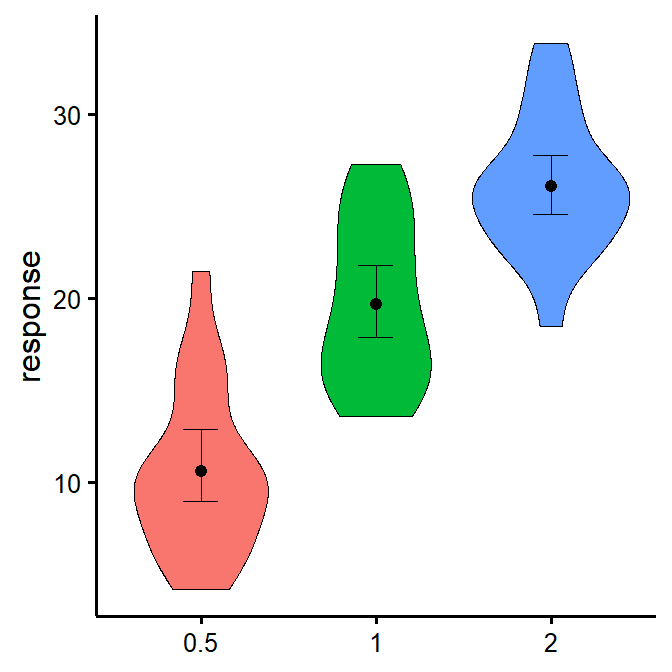Dots  = Means
Error bars = 95% bootstrapped confidence Intervals (2000 replications)
Width = Distribution Density (Frequency)

This is cool because it gives you a 95% bootstrapped confidence interval (with 2000 bootstraps), as shown by the error bars. Bootstrapping is a non-parametric technique, meaning that it does not need to respect the classical parametric assumptions (normality, homoscedasticity, etc.). In this case it’s just a nice alternative way to look at your data.

Note: bootstrapping requires a number of bootstraps equal or higher than your number of observations (rows). Because people sometimes work with big datasets, I’ve added an option to specify a specific number of bootstraps with the option bootstraps = 2000 (change default to your desired value). But because I’ve recently had to work with a very large data set (7 million observations), it was impractical to do 7 million bootstraps (that would have been a very, very long operation), so I also added an option to turn bootstrapping off completely and just use a regular confidence interval instead; just add boot = FALSE as one of the argument.

### Save a high-resolution image file to specified directory

ggsave('niceviolinplothere.tiff', width = 7, height = 7, unit = 'in', dpi = 300, path = "~")
# This will save to, e.g., "C:/Users/Username/Documents/".
# You can change the path to where you would like to save it.
# If you do change the path manually, remember to use "R" slashes ('/' rather than '\').
# Also remember to specify the .tiff extension of the file.

Pro tip: Change .tiff for .pdf or .eps for scalable vector graphics for high-resolution submissions to scientific journals!

$$~$$

### Change x- and y- axes labels

niceViolin(group = factor(ToothGrowth$dose), response = ToothGrowth$len,
ytitle = "Length of Tooth",
xtitle = "Vitamin C Dosage")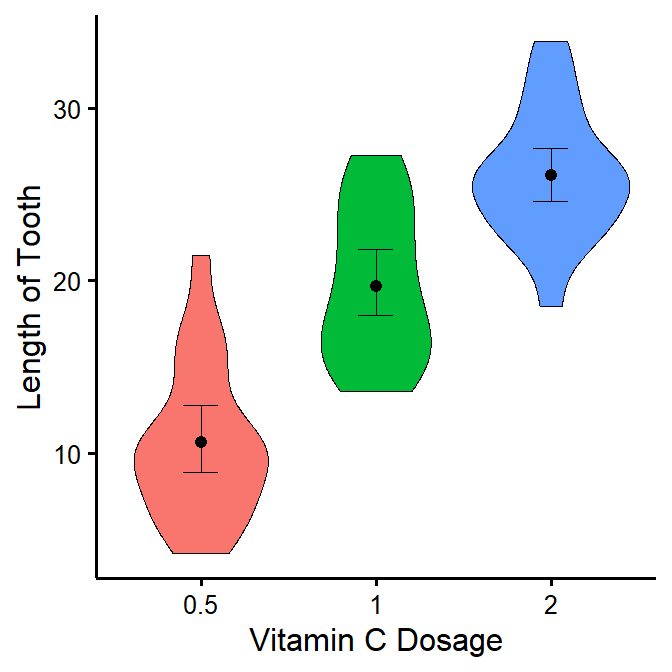### See difference between two groups

To see if two groups are statistically significantly different.

niceViolin(group = factor(ToothGrowth$dose), response = ToothGrowth$len,
comp1 = "0.5",
comp2 = "2")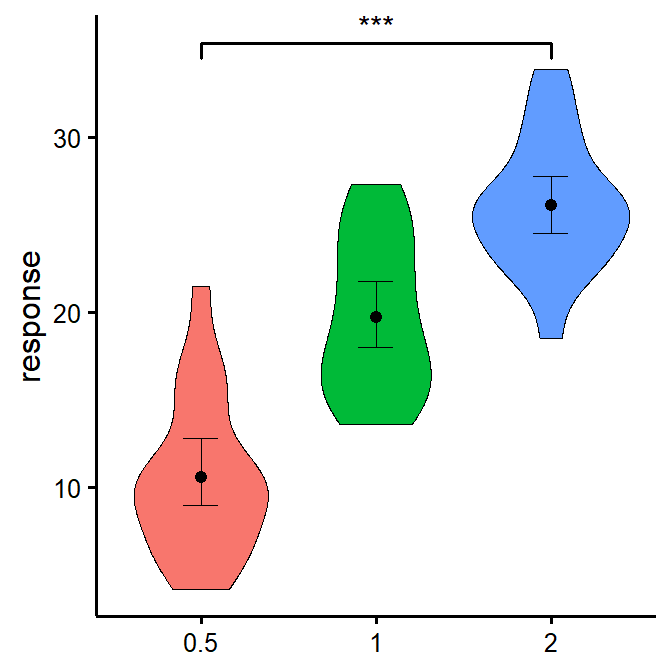### See difference between two other groups

You can also select groups based on their position on the x-axis (notice no quotes this time).

niceViolin(group = factor(ToothGrowth$dose), response = ToothGrowth$len,
comp1 = 2,
comp2 = 3)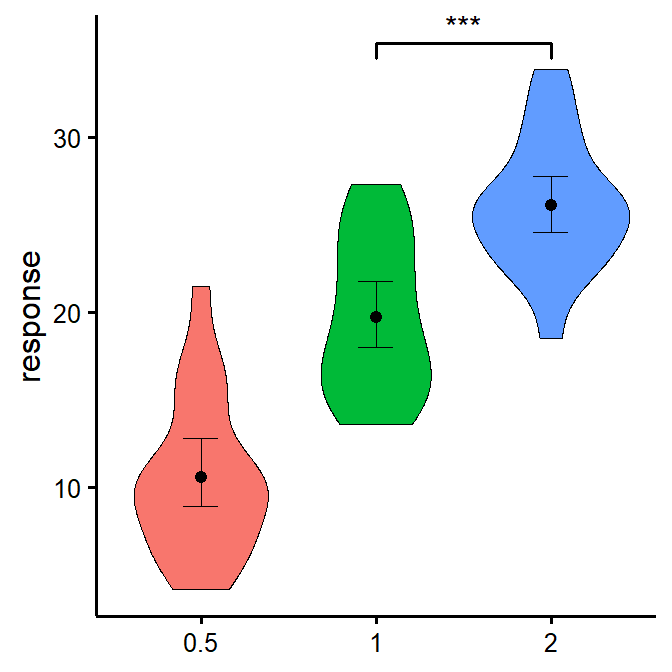### Compare all three groups

What if you want to look at all three groups at the same time? Unfortunately, the underlying package we use, ggsignif, does not allow the comparison of more than one group at once. So we need to tweak this manually instead. (Note that we can also use this technique when the significance computed with ggsignif does not correspond to the number of stars we want to use, for example if we use a different p-value or not a t-test.)

niceViolin(group = factor(ToothGrowth$dose), response = ToothGrowth$len,
signif_annotation = c("*","**","***"), # manually enter the number of stars
signif_yposition = c(30,35,40), # At what values of y the stars should appear (vertically)
signif_xmin = c(1,2,1), # At what values of x stars should appear (left-sided bracket)
signif_xmax = c(2,3,3)) # At what values of x stars should appear (right-sided bracket)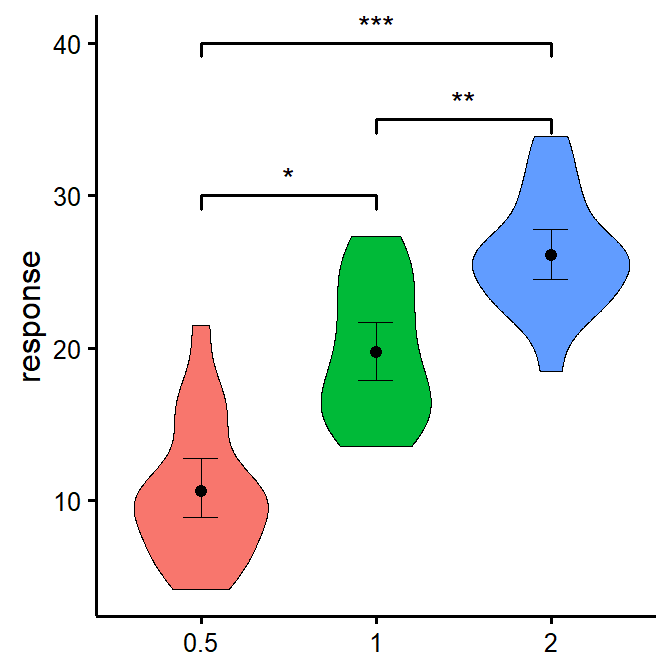### Set the colours manually

niceViolin(group = factor(ToothGrowth$dose), response = ToothGrowth$len,
colours = c("darkseagreen","cadetblue","darkslateblue"))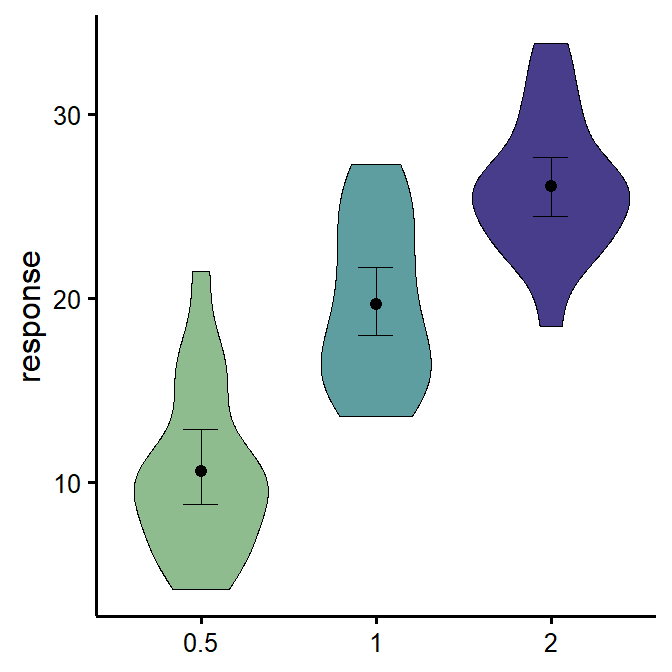### Changing the names of the x-axis labels

niceViolin(group = factor(ToothGrowth$dose), response = ToothGrowth$len,
xlabels = c("Low", "Medium", "High"))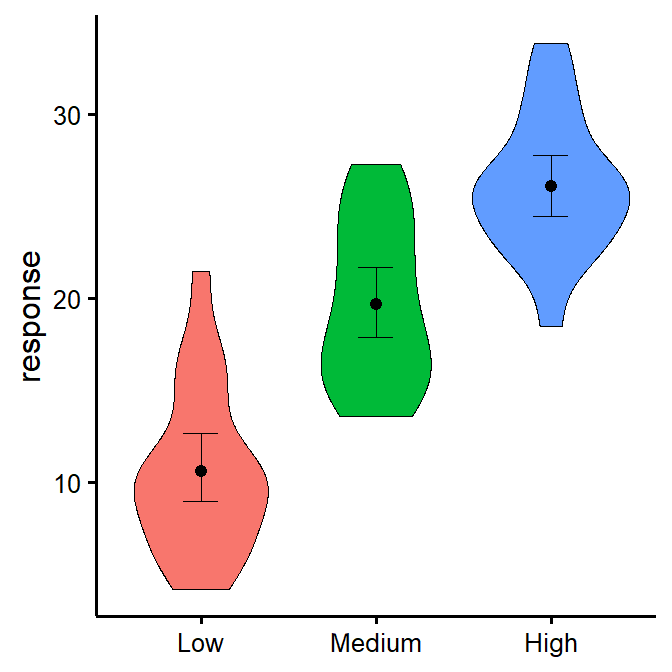### Removing the x-axis or y-axis titles

niceViolin(group = factor(ToothGrowth$dose), response = ToothGrowth$len,
ytitle = NULL,
xtitle = NULL)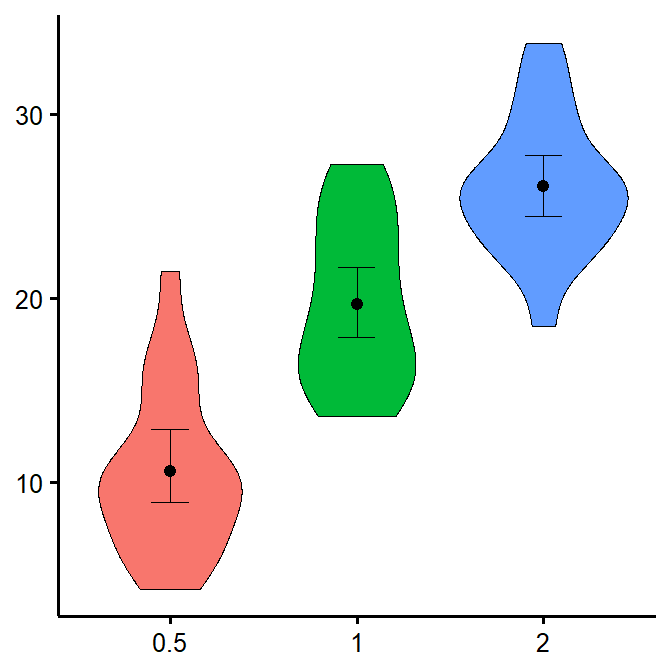### Removing the x-axis or y-axis labels (for whatever purpose)

niceViolin(group = factor(ToothGrowth$dose), response = ToothGrowth$len,
has.ylabels = FALSE,
has.xlabels = FALSE)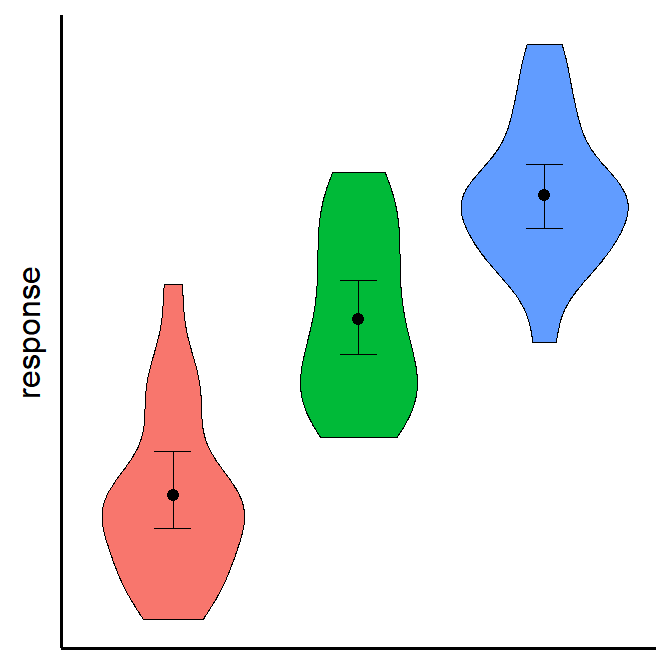### With x number of groups

x = 6
niceViolin(group = factor(sample(1:x, 60, replace=T)),
response = ToothGrowth$len)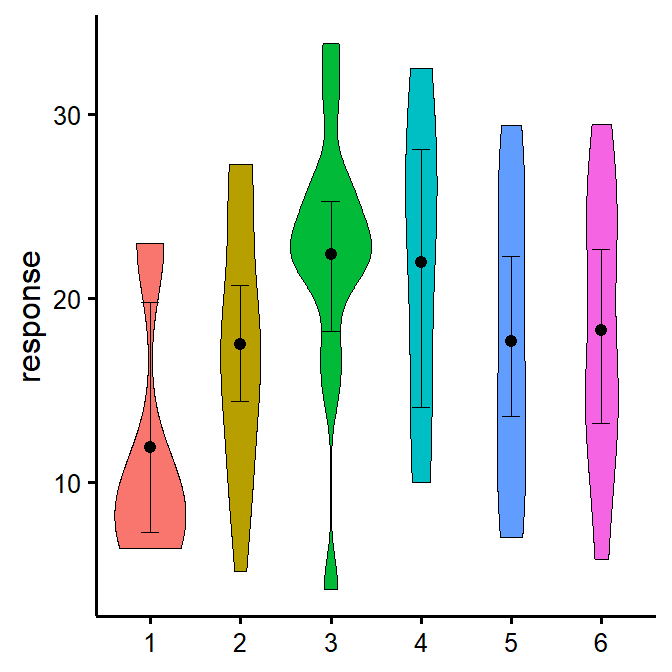Pro tip: Save figure with greater width when you have more groups! $$~$$ ### Putting it all together If you’d like to see all available options at once (a bit long): niceViolin(group = factor(sample(1:4,60, replace=T)), response = ToothGrowth$len,
ytitle = "Length of Tooth",
xtitle = "Vitamin C Dosage",
colours = c("darkseagreen", "cadetblue", "darkslateblue", "deeppink4"),
has.ylabels = TRUE,
has.xlabels = TRUE,
xlabels = c("None", "Low", "Medium", "High"),
comp1 = NULL,
comp2 = NULL,
signif_annotation = c("NS","*","**","***"),
signif_yposition = c(35,40,35,45),
signif_xmin = c(1,2,3,1),
signif_xmax = c(2,3,4,4))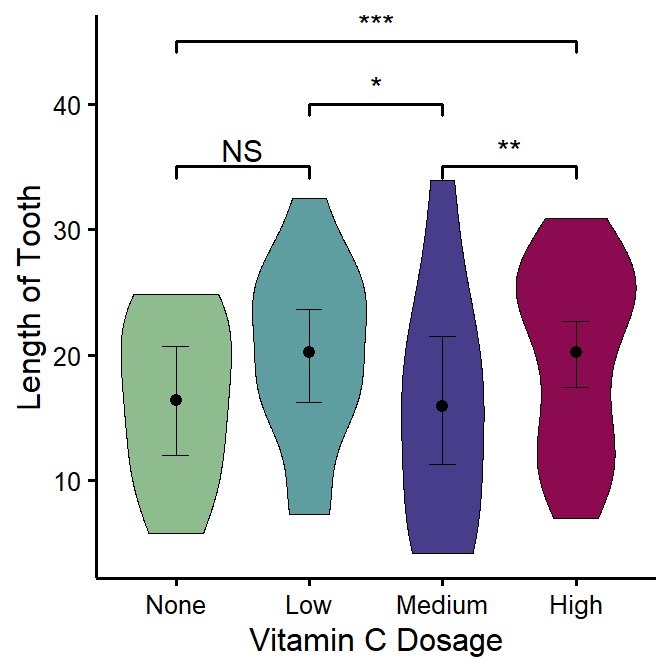## Special situation: Add other plot elements

The good thing about this function is that it outputs a ggplot object, which you can continue to use to build on your plot and customize it futher. For instance, I recently wanted to add mean and sample size as annotations for each group (where I had group sample sizes ranging from 100 to 35,000, so it was important to know which groups were more representative). For the demonstration, let’s just build the same multiple-groups plot we did earlier.

# Create our group variable:
groups <- factor(sample(1:6,60, replace=T))

# Make the plot and save it to object "p"
p <- niceViolin(group = groups,
response = ToothGrowth$len) Then what I did is that I simply added conventional ggplot code to my plot object. (But first I had to compute the statistics we were going to be using:) # Compute basic statistics and save to object library(psych) # Install the psych package if you don't already have it statsSummary <- describeBy(x = ToothGrowth$len, group = groups, mat = TRUE)

p + annotate(geom="text", # First annotation adds the average
x=1:length(levels(groups)), # Specifies annotations is for all groups/x-axis ticks
y=statsSummary$mean+2, # Puts mean at mean value on the y-axis (adds 2) label=paste0("m=", round(statsSummary$mean,2))) + # That prints the mean on the plot
annotate(geom="text", # (Second annotation adds the sample size)
x=1:length(levels(groups)),
y=statsSummary$mean-2, # Puts sample size at mean value on the y-axis (substracts 2) label=paste0("n=", round(statsSummary$n,2))) # That prints the sample size on the plot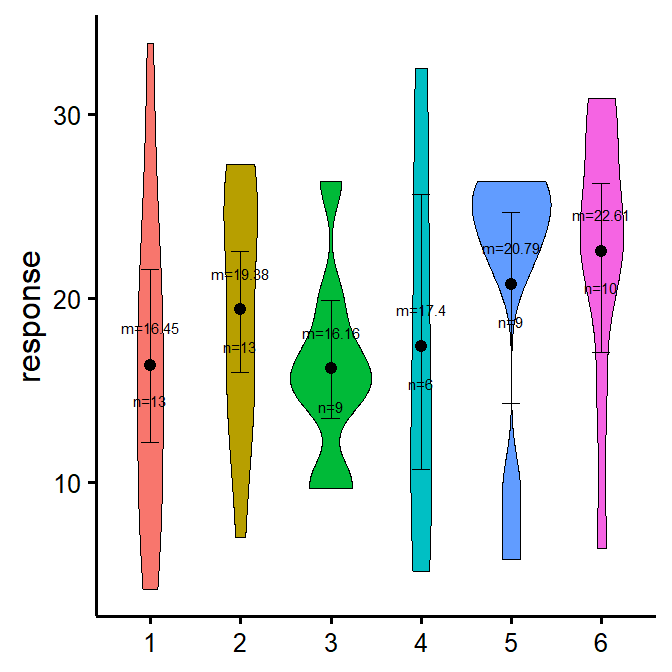$$~$$

$$~$$

### Concluding Statement

Make sure to check out this page again if you use the code after a time or if you encounter errors, as I periodically update or improve the code.

You can always edit the function to suit your purposes, or contact me for questions or requests to modify this function at remitheriault.wixsite.com/site/contact! Thanks for reading my guide! :) $$~$$

$$~$$

$$~$$

$$~$$

$$~$$

Updated 2020-05-29

$$~$$

$$~$$

$$~$$

$$~$$

$$~$$

$$~$$